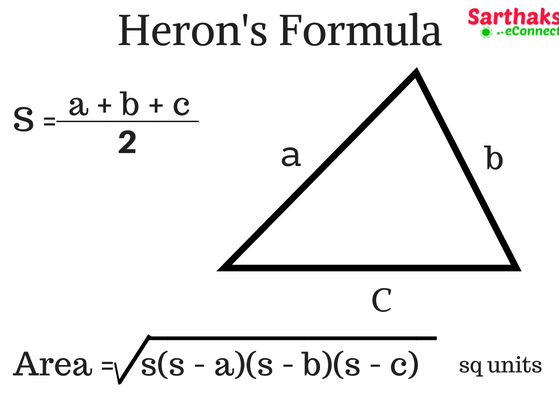# Heron’s Formula view

How surprising it is that for finding the area of triangle we should not have to use various measurements like altitude or else. We can calculate area of triangle by simply its side or by its perimeter by the use of Heron’s formula.

It is applicable to every type of triangles. It can also be used in calculating area of quadrilateral as quadrilateral can be reduced to two triangles by joining any one diagonals.

## Area of triangle:

Area of triangle = $$\frac{1}{2}$$ x base x height

This calculation is easy in case of right triangle as simply we have to multiply its base side and perpendicular side and divide it by 2. But for the rest it becomes difficult. So heron’s formula is preferred to calculate area of those triangles.

## Heron’s Formula:Heron was a scientist and mathematician of 10 AD. His works on mathematical and physical subjects are so numerous and varied that he is considered to be an encyclopedic writer in these fields.

Heron’s formula is named after him and is stated as

Area = $$\sqrt{s(s-a)(s-b)(s-c)}$$

S = $$\frac{perimeter}{2}$$ = $$\frac{a\:+\:b\:+\:c}{2}$$

Where a, b, c are sides of the triangle.

S is semi-perimeter.

## Area of quadrilateral by Heron’s formula:

As we discussed earlier that this formula has a lot of application in solving area of quadrilateral. After reducing a quadrilateral into to triangles and measuring its sides we can calculate area of quadrilateral.

## Heron’s formula examples:

1. Two sides of a triangle are 8 cm and 11 cm and the perimeter is 32 cm. Find the area of a triangle.Solution:

As there two sides of triangle and perimeter is given then we can find its 3rd side.
a= 8cm, b = 11cm, c = 32 – (11 + 8) = 13cm
Now, Semi-perimeter
S = $$\frac{perimeter}{2}$$
S = $$\frac{32}{2}$$ = 16
Area = $$\sqrt{s(s-a)(s-b)(s-c)}$$
Area = $$\sqrt{16(16-8)(16-11)(16-13)}$$ = 8$$\sqrt{30}$$ cm2

1. The sides of a triangular plot are in the ratio of 3 : 5 : 7 and its perimeter is 300 m. Find its area.Solution:

Let the ratio constant be x
so sides are a = 3x, b = 5x, c = 7x
A/q 3x + 5x +7x = 300
: x = 20
a = 60m , b = 100m, c = 140m
Semi-perimeter = $$\frac{300}{2}$$ = 150m
Now, for area we apply heron’s formula
$$\sqrt{s(s-a)(s-b)(s-c)}$$
Area = $$\sqrt{150(150-60)(150-100)(150-140)}$$ = 1500$$\sqrt{3}$$ m2